# Right now writing detailed YOLO v3 tutorials for TensorFlow 2.x

Detection and custom training process works better, is more accurate and has more planned features to do:
https://github.com/pythonlessons/TensorFlow-2.x-YOLOv3

# TensorFlow 1.* Object Detection Introduction

### Original text version of tutorial you can visit here.

The purpose of this tutorial is to learn how to install and prepare TensorFlow framework to train your own convolutional neural network object detection classifier for multiple objects, starting from scratch. At the end of this tutorial, you will have basics and a program that can identify and draw boxes around specific objects in computer screen.

There are several good tutorials available for how to use TensorFlow’s Object Detection API to train a classifier for a single or several objects, but they are not that detailed how we want they to be. More over Object Detection API seems to have been developed on a Linux-based OS. To set up TensorFlow to train and use a model on Windows, there are several workarounds that need to be used in place of commands that would work fine on Linux. Also, this tutorial provides instructions for training a classifier that can detect multiple objects, not just one.

This tutorial is written and tested for Windows 10 operating system, it should also work for Windows 7 and 8, but haven’t tested that, so I am not sure. The general procedure can also be used for Linux operating systems, but file paths and package installation commands should be changed accordingly.

TensorFlow-GPU allows your PC to use the video card to provide extra processing power while training, so it will be used for this tutorial. In my experience, using TensorFlow-GPU instead of regular TensorFlow reduces training time by a factor of about 8 (depends on used CPU and trained model).

Regular CPU version TensorFlow can also be used for this tutorial, but it will take longer and real time models may work slower. If you use regular TensorFlow, you do not need to install CUDA and cuDNN in installation step. I used newest TensorFlow-GPU v1.11 while creating this tutorial, but it also should work for future versions of TensorFlow, but I am not guaranteed.

Vision of this tutorial: to create TensorFlow object detection model, that could detect CS:GO players. In this tutorial we’ll be more focused on detecting terrorists and counter terrorists bodies and heads, then automatically aim to them and shoot. At first, it seems like an easy task, but going through this tutorial series you will see that there is more problems than it seems.

Main computer specs for this tutorial:

• OS – Windows 10 Pro
• GPU - GTX1080TI
• CPU – i5-6400
• RAM – 16GB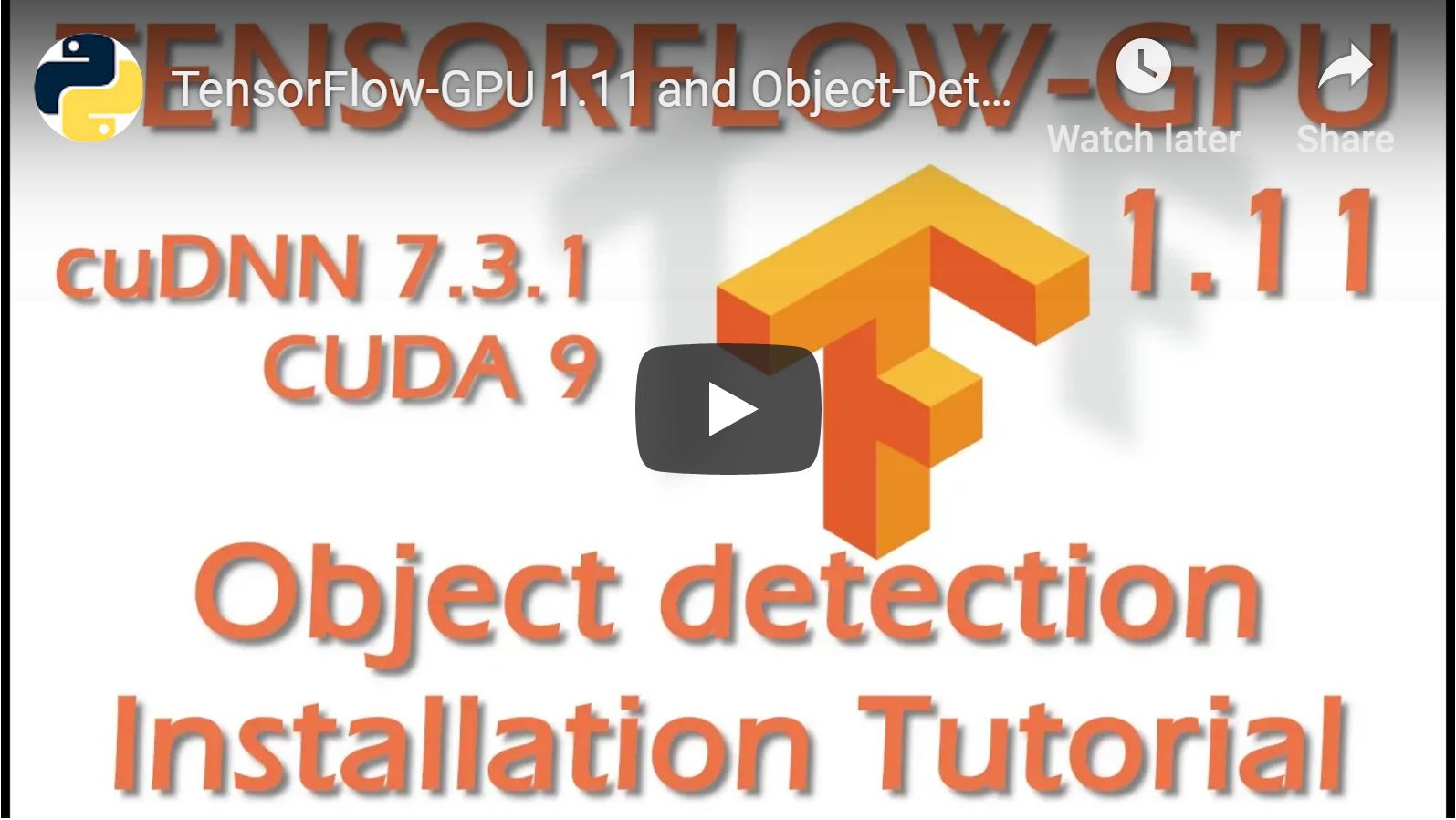## TensorFlow-GPU 1.11, CUDA v9.0, cuDNN v7.3.1 installation

Hardware requirements:

• NVIDIA® GPU card with CUDA® Compute Capability 3.5 or higher
• Strong enough computer (high end CPU and at least 8GB of RAM)

Software requirements:

In many places there was said that there is some problems while working on newest CUDA versions, but I took this challenge and installed CUDA v10.0 and cuDNN v7.3.1. As future versions of TensorFlow will be released, you will likely need to continue updating the CUDA and cuDNN versions to the latest supported version. If you face problems while installing CUDA, visit this documentation site. If you face problems while installing cuDNN, visit this documentation site. This tutorial is made for TensorFlow-GPU v1.11, so the “pip install tensorflow-gpu” command should automatically download and install newest 1.11 version.

## Install Python 3.6 64-bit version and install all required packages

If you don’t know how to install python, follow my tutorial on PyLessons page. 32-bit version doesn’t support TensorFlow so don’t even try. If you already have python installed, install required packages:

``````pip install pillow
pip install lxml
pip install Cython
pip install jupyter
pip install matplotlib
pip install pandas
pip install opencv-python
pip install tensorflow-gpu
``````

## Set up TensorFlow Object Detection repository

This working directory will contain the full TensorFlow object detection framework, as well as your training images, training data, trained classifier, configuration files, and everything else needed for the object detection classifier.

You can follow my tutorial with installation and repository preparation or follow original TensorFlow tutorial (Must mention it didn’t worked for me with official tutorial).

When you already have TensorFlow models on your disk, you must add object detection directories to python path (if it doesn’t work from CMD line, do it manually like I did on video tutorial):

Configure PYTHONPATH environment variable (in my case):

``````set PYTHONPATH=\$PYTHONPATH;C:\TensorFlow \research
set PYTHONPATH=\$PYTHONPATH;C:\TensorFlow \research\slim
``````

Next, compile the Protobuf files, which are used by TensorFlow to configure model and training parameters. Unfortunately, the short protoc compilation command posted on TensorFlow’s Object Detection API installation page doesn’t work for me on Windows. So I downloaded older 3.4.0 version of protoc from here. Transfered files to same TensorFlow directory and ran the following command from the tensorflow /research/ directory:

``````# From tensorflow/research/
"C:/TensorFlow/bin/protoc" object_detection/protos/*.proto --python_out=.
``````

Finally, run the following command from the C:\ TensorFlow\research directory:

``````python setup.py install
``````

You can test that you have correctly installed the Tensorflow Object Detection API by running the following command:

``````# From tensorflow/research/object_detection
python builders/model_builder_test.py
``````

If everything was fine you can test it out and verify your installation is working by launching the object_detection_tutorial.ipynb script with Jupyter. From the object_detection directory, issue this command:

``````# From tensorflow/research/object_detection
jupyter notebook
``````

In your browser click on „object_detection_tutorial.ipynb“. Then navigate to „Cell“ in navigation bar and click on „Run All“. If everything is fine in short time you should see these nice photos: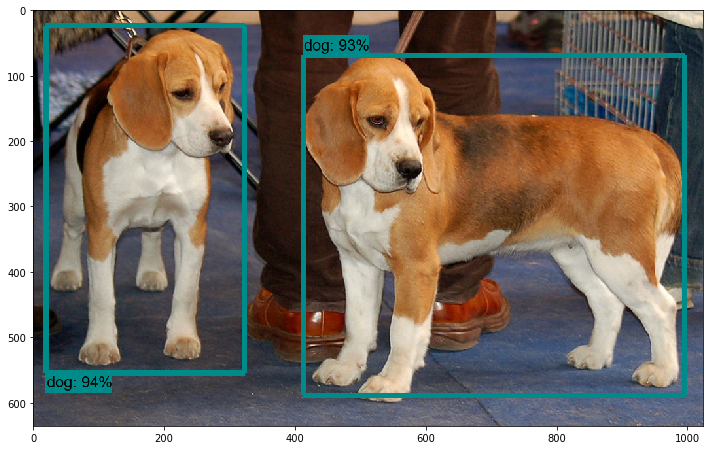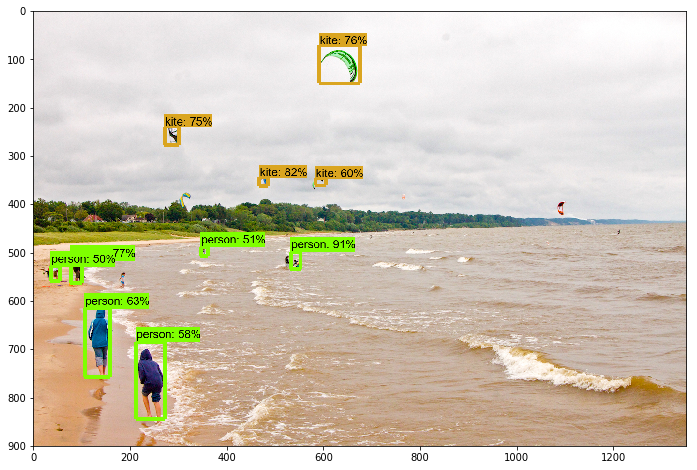# Part 1. TensorFlow Object Detection merged with grabscreen

In this part we are going to merge jupyter API code from 1-st tutorial with code from 2-nd tutorial where we tested 3 different ways of grabbing screen.

To begin, we're going to modify the notebook first by converting it to a .py file. If you want to keep it in a notebook, that's fine too. To convert, you can go to file > download as > python file. Once that's done, we're going to comment out the lines we don't need.

Once you have your converted object detection file, go to your TensorFlow installation folder: research\object_detection\data and grab mscoco_label_map.pbtxt file, place it to you working directory.

Next you should download pretrained model from here, I am using faster_rcnn_inception_v2_coco, so I recommend you to use the same, at least at the beginning. Take frozen_inference_graph.pb file and transfer it to your local working repository.

So we begin by importing time, CV2, MSS libraries. If you don't have them, install before moving forward.

I personally imported line to disable CUDA devices, because I wanted to run this example on CPU, because running tensorflow-gpu takes more time to startup in backend.

``````os.environ['CUDA_VISIBLE_DEVICES'] = '-1'
``````

Then we don't need to import tarfile and import zipfile, because we are not working with these files, so we comment them out now. Going further we comment `#from matplotlib import pyplot as plt` and `#from PIL import Image` lines, because we are doing things our way.

Next I am importing few lines from my second tutorial for grabing screen and measuring FPS:

``````# title of our window
title = "FPS benchmark"
# set start time to current time
start_time = time.time()
# displays the frame rate every 2 second
display_time = 2
# Set primarry FPS to 0
fps = 0
# Load mss library as sct
sct = mss.mss()
# Set monitor size to capture to MSS
monitor = {"top": 40, "left": 0, "width": 800, "height": 640}
``````

Because we are not using notebook anymore we are not using and these lines:

``````#sys.path.append("..")

#if StrictVersion(tf.__version__) < StrictVersion('1.9.0'):
``````

We are not using matplotlib to display image, so we are commenting line used for that:

``````#get_ipython().run_line_magic('matplotlib', 'inline')
``````

There are two lines of import before going to an actual code:

``````from utils import label_map_util
from utils import visualization_utils as vis_util
``````

But if you will try to use them like this, you will get an error, so add `object_detection.` before utils, just like this:

``````from object_detection.utils import label_map_util
from object_detection.utils import visualization_utils as vis_util
``````

Next is links to paths, if you would like to have everything in same folder, just like in my tutorial, comment all these lines:

``````# What model to download.
MODEL_NAME = 'ssd_mobilenet_v1_coco_2017_11_17'
MODEL_FILE = MODEL_NAME + '.tar.gz'

# Path to frozen detection graph. This is the actual model that is used for the object detection.
PATH_TO_FROZEN_GRAPH = MODEL_NAME + '/frozen_inference_graph.pb'

# List of the strings that is used to add correct label for each box.
PATH_TO_LABELS = os.path.join('data', 'mscoco_label_map.pbtxt')
``````

And replace them with my used path lines (don't forget to add NUM_CLASSES = 99 line)

``````MODEL_NAME = 'inference_graph'
PATH_TO_FROZEN_GRAPH = 'frozen_inference_graph.pb'
PATH_TO_LABELS = 'mscoco_label_map.pbtxt'
NUM_CLASSES = 99
``````

Next you can comment all  part, because we won't use it:

``````#opener = urllib.request.URLopener()
#tar_file = tarfile.open(MODEL_FILE)
#for file in tar_file.getmembers():
#  file_name = os.path.basename(file.name)
#  if 'frozen_inference_graph.pb' in file_name:
#    tar_file.extract(file, os.getcwd())
``````

Next, add 3: label_map, categories and category_index lines before detection_graph code:

``````label_map = label_map_util.load_labelmap(PATH_TO_LABELS)
categories = label_map_util.convert_label_map_to_categories(label_map, max_num_classes=NUM_CLASSES, use_display_name=True)
category_index = label_map_util.create_category_index(categories)
``````

and in part  comment or delete category_index line:

``````category_index = label_map_util.create_category_index_from_labelmap(PATH_TO_LABELS, use_display_name=True)
``````

Comment all lines in part  where images were loaded to numpy array:

``````#def load_image_into_numpy_array(image):
#  (im_width, im_height) = image.size
#  return np.array(image.getdata()).reshape(
#      (im_height, im_width, 3)).astype(np.uint8)
``````

Next you can delete PATH_TO_TEST_IMAGES_DIR, TEST_IMAGE_PATHS and IMAGE_SIZE lines, but if you will leave them it wound effect our code:

``````PATH_TO_TEST_IMAGES_DIR = 'test_images'
TEST_IMAGE_PATHS = [ os.path.join(PATH_TO_TEST_IMAGES_DIR, 'image{}.jpg'.format(i)) for i in range(1, 3) ]
IMAGE_SIZE = (12, 8)
``````

At the end of code, not to make any mistakes you can replace all  block code with this code:

``````with detection_graph.as_default():
with tf.Session(graph=detection_graph) as sess:
while True:
# Get raw pixels from the screen, save it to a Numpy array
image_np = np.array(sct.grab(monitor))
# to ger real color we do this:
image_np = cv2.cvtColor(image_np, cv2.COLOR_BGR2RGB)
#image = Image.open(image_path)
# the array based representation of the image will be used later in order to prepare the
# result image with boxes and labels on it.
# Expand dimensions since the model expects images to have shape: [1, None, None, 3]
image_np_expanded = np.expand_dims(image_np, axis=0)
# Actual detection.
output_dict = run_inference_for_single_image(image_np, detection_graph)
# Visualization of the results of a detection.
vis_util.visualize_boxes_and_labels_on_image_array(
image_np,
output_dict['detection_boxes'],
output_dict['detection_classes'],
output_dict['detection_scores'],
category_index,
use_normalized_coordinates=True,
line_thickness=8)
#plt.figure(figsize=IMAGE_SIZE)
#plt.imshow(image_np)
cv2.imshow(title, cv2.cvtColor(image_np, cv2.COLOR_BGR2RGB))
fps+=1
TIME = time.time() - start_time
if (TIME) >= display_time :
print("FPS: ", fps / (TIME))
fps = 0
start_time = time.time()
# Press "q" to quit
if cv2.waitKey(25) & 0xFF == ord("q"):
cv2.destroyAllWindows()
break
``````

So I tried to use this slow object detection method on image where you can see crowd of people walking across the street: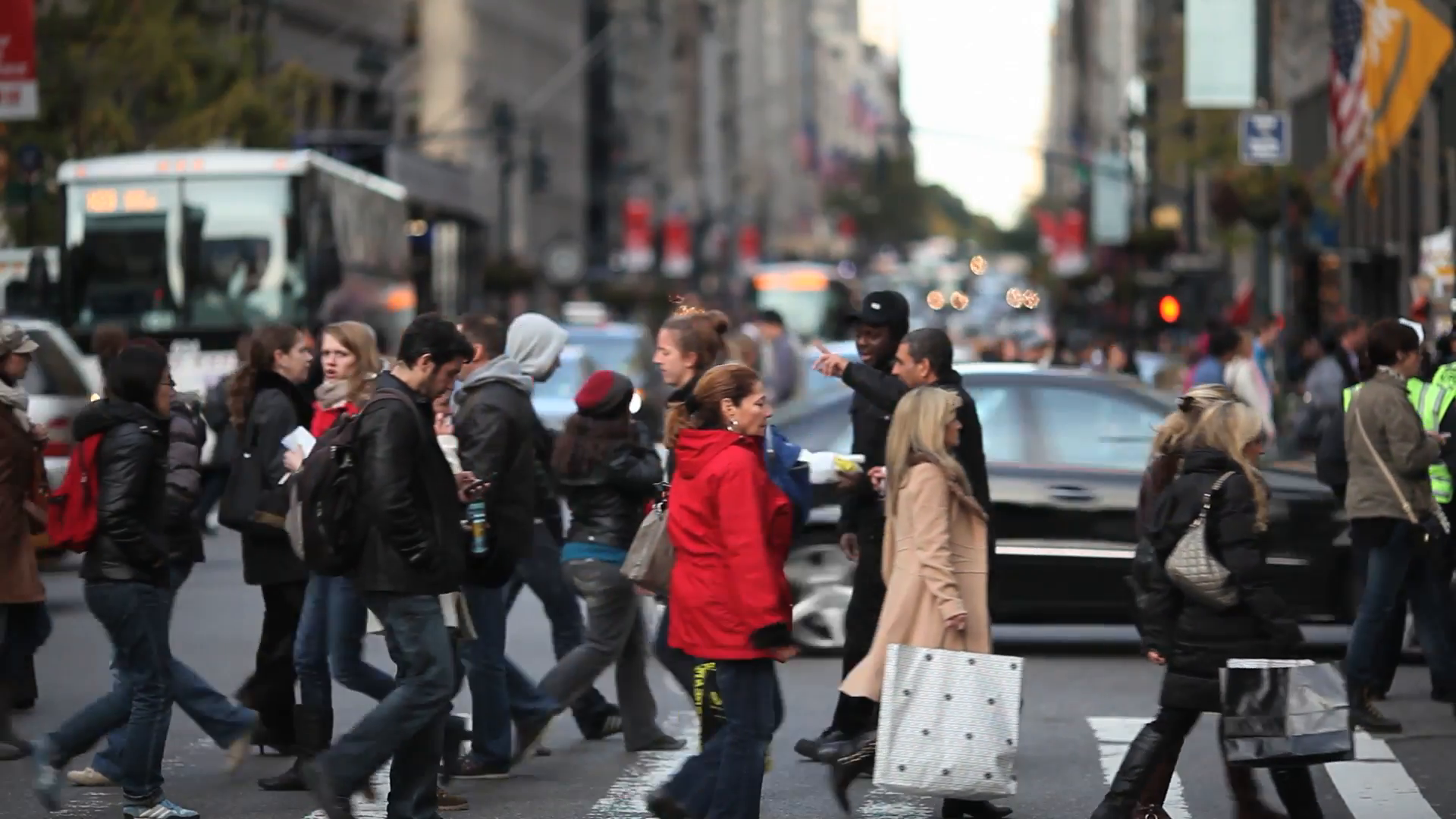And here is the results of frames per second working with TensorFlow CPU version. In average, it is about 7 seconds to receive one frame per second. So if we would like to use it for real time purpose, this would be impossible to do something useful with it. So we need to make it work much faster, we are doing so in second part below.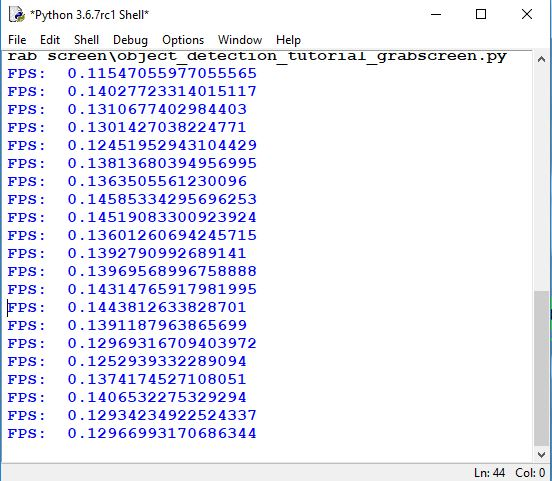# Part 2. TensorFlow Object Detection merged with grabscreen

### Original text version of tutorial you can visit here.

In previous tutorial we ran actual pretrained object detection, but our code is messy and detection was working really slow. In this part we are cleaning the messy code and making some code modifications that our object detection would work in much faster way.

At first I went through all code and deleted all unecassary code, so instead of using `object_detection_tutorial_grabscreen.py`, better take `object_detection_tutorial_grabscreen_pretty.py` it will be much easier to understand how it works.

After cleaning the code, I started to make some changes to it. Mainly what I done is that I deleted `def run_inference_for_single_image(image, graph):` function and added needed lines to main while loop, and this changed object detection speed. Not taking into details I will upload code part I changed:

``````# # Detection
with detection_graph.as_default():
with tf.Session(graph=detection_graph) as sess:
while True:
# Get raw pixels from the screen, save it to a Numpy array
image_np = np.array(sct.grab(monitor))
# To get real color we do this:
image_np = cv2.cvtColor(image_np, cv2.COLOR_BGR2RGB)
# Expand dimensions since the model expects images to have shape: [1, None, None, 3]
image_np_expanded = np.expand_dims(image_np, axis=0)
# Actual detection.
image_tensor = detection_graph.get_tensor_by_name('image_tensor:0')
boxes = detection_graph.get_tensor_by_name('detection_boxes:0')
scores = detection_graph.get_tensor_by_name('detection_scores:0')
classes = detection_graph.get_tensor_by_name('detection_classes:0')
num_detections = detection_graph.get_tensor_by_name('num_detections:0')
# Visualization of the results of a detection.
(boxes, scores, classes, num_detections) = sess.run(
[boxes, scores, classes, num_detections],
feed_dict={image_tensor: image_np_expanded})
vis_util.visualize_boxes_and_labels_on_image_array(
image_np,
np.squeeze(boxes),
np.squeeze(classes).astype(np.int32),
np.squeeze(scores),
category_index,
use_normalized_coordinates=True,
line_thickness=3)
# Show image with detection
cv2.imshow(title, cv2.cvtColor(image_np, cv2.COLOR_BGR2RGB))
# Bellow we calculate our FPS
fps+=1
TIME = time.time() - start_time
if (TIME) >= display_time :
print("FPS: ", fps / (TIME))
fps = 0
start_time = time.time()
# Press "q" to quit
if cv2.waitKey(25) & 0xFF == ord("q"):
cv2.destroyAllWindows()
break
``````

Same as in 3-rd part, we are testing how fast it is working. To compare results we got in 3-rd tutorial part we are taking the same picture, with the same object count in it. In bellow image, you can see significant difference comparing what we had before, it is in average 1 FPS. If you will run it on GPU you will get from 5 to 10 times boost.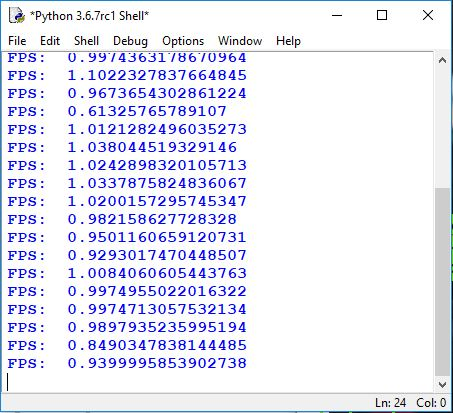# Part 3. TensorFlow Object Detection step by step custom object detection tutorial

Welcome to part 5 of the TensorFlow Object Detection API tutorial series. In this part and few in future, we're going to cover how we can track and detect our own custom objects with this API.

I am doing this by using the pre-built model to add custom detection objects to it. That’s a decent jump from my findings, and it’s quite hard to locate any full step-by-step tutorials, so hopefully I can help you with that. Once you finish this tutorial you will have the ability to train for any custom object you can think of (and create data for) - that’s an awesome skill to have in my opinion.

Alright, so this is my overview of the steps needed to do in this tutorial:

• Collect at least 500 images that contain your object - The bare minimum would be about 100, ideally more like 1000 or more, but, the more images you have, the more tedious step 2 will be.
• Annotate/label the images. I am personally using LabelImg. This process is basically drawing boxes around your object(s) in an image. The label program automatically will create an XML file that describes the object(s) in the pictures.
• Split this data into train/test samples. Training data should be around 80% and testing around 20%.
• Generate TF Records from these splits.
• Setup a .config file for the model of choice (you could train your own from scratch, but we'll be using transfer learning).
• Train our model.
• Export inference graph from new trained model.
• Detect custom objects in real time.

TensorFlow needs hundreds of images of an object to train a good detection classifier, best would be at least 1000 pictures for one object. To train a robust classifier, the training images should have random objects in the image along with the desired objects, and should have a variety of backgrounds and lighting conditions. There should be some images where the desired object is partially obscured, overlapped with something else, or only halfway in the picture.

In my classifier, I will use four different objects I want to detect (terrorist, terrorist head, counter-terrorist and counter-terrorist head). I used to play CSGO with harmless bots to gather hundreds of these images playing on few different game maps, also I was grabbing some pictures from google, to make them different from my game.

### Label Pictures:

Here comes the best part. With all the pictures gathered, it’s time to label the desired objects in every picture. LabelImg is a great tool for labeling images, and its GitHub page has very clear instructions on how to install and use it.

Download and install LabelImg, when running this, you should get a GUI window. From here, choose to open dir and pick the directory that you saved all of your images to. Now, you can begin to annotate images with the create rectbox button. Draw your box, add the name in, and hit ok. Save, hit next image, and repeat! You can press the w key to draw the box and do ctrl+s to save faster. For me it took in average 1 hour for 100 images, this depends on object quantity you have in image. Keep in mind, this will take a while!

LabelImg saves a .xml file containing the label data for each image. These .xml files will be used to generate TFRecords, which are one of the inputs to the TensorFlow trainer. Once you have labeled and saved each image, there will be one .xml file for each image in the `\test` and `\train` directories.

Once you have labeled your images, we're going to separate them into training and testing groups. To do this, just copy about 20% of your images and their annotation XML files to a new dir called test and then copy the remaining ones to a new dir called train.

With the images labeled, it’s time to generate the TFRecords that serve as input data to the TensorFlow training model. This tutorial uses the xml_to_csv.py and generate_tfrecord.py scripts from EdjeElectronics repository, with some slight modifications to work with our directory structure.

First, the image .xml data will be used to create .csv files containing all the data for the train and test images. From the main folder, if you are using the same file structure issue the following command in command prompt: `python xml_to_csv.py`.

This creates a train_labels.csv and test_labels.csv file in the CSGO_images folder. If you are using different files structure, please change `xml_to_csv.py` accordingly. To avoid of using cmd I created short .bat script called `xml_to_csv.bat`.

Next, open the generate_tfrecord.py file in a text editor, this file I also took from EdjeElectronics repository. Replace the label map with your own label map, where each object is assigned with an ID number. This same number assignment will be used when configuring the `labelmap.pbtxt` file.

For example, if you are training your own classifier, you will replace the following code in `generate_tfrecord.py`:

``````# TO-DO replace this with label map
def class_text_to_int(row_label):
if row_label == 'c':
return 1
elif row_label == 'ch':
return 2
elif row_label == 't':
return 3
elif row_label == 'th':
return 4
else:
return None
``````

Then, generate the TFRecord files by starting my created `generate_tfrecord.bat` file, which is issuing these commands from local folder:

``````python generate_tfrecord.py --csv_input=CSGO_images\train_labels.csv --image_dir=CSGO_images\train --output_path=CSGO_images\train.record
python generate_tfrecord.py --csv_input=CSGO_images\test_labels.csv --image_dir=CSGO_images\test --output_path=CSGO_images\test.record
``````

These lines generates a `train.record` and a `test.record` files in training folder. These will be used to train the new object detection classifier.

The last thing to do before training is to create a label map and edit the training configuration file. The label map tells the trainer what each object is by defining a mapping of class names to class ID numbers. Use a text editor to create a new file and save it as `labelmap.pbtxt` in the training folder. (Make sure the file type is `.pbtxt`). In the text editor, copy or type the label map in the same format as below (the example below is the label map for my CSGO detector). The label map ID numbers should be the same as defined in the `generate_tfrecord.py` file.

``````item {
id: 1
name: 'c'
}

item {
id: 2
name: 'ch'
}

item {
id: 3
name: 't'
}

item {
id: 4
name: 'th'
}
``````

### Configure training:

Finally, the object detection training pipeline must be configured. It defines which model and what parameters will be used for training. This is the last step before running actual training.

Navigate to your TensorFlow `research\object_detection\samples\configs` directory and copy the `faster_rcnn_inception_v2_coco.config` file into the CSGO_training directory. Then, open the file with a text editor, I personally use notepad++. There are needed several changes to make to this `.config` file, mainly changing the number of classes, examples and adding the file paths to the training data.

By teh way, the paths must be entered with single forward slashes "`/`", or TensorFlow will give a file path error when trying to train the model. The paths must be in double quotation marks ( `"` ), not single quotation marks ( `'` ).

`Line 10.` Change num_classes to the number of different objects you want the classifier to detect. For my CSGO object detection it would be num_classes : 4
`Line 107.` Change fine_tune_checkpoint to:
fine_tune_checkpoint : "faster_rcnn_inception_v2_coco_2018_01_28/model.ckpt"
`Lines 122 and 124.` In the train_input_reader section, change input_path and label_map_path to:
input_path: "CSGO_images/train.record"
label_map_path: "CSGO_training/labelmap.pbtxt"
`Line 128.` Change num_examples to the number of images you have in the CSGO_images\test directory. I have 113 images, so I change it to: "num_examples: 113"
`Lines 136 and 138.` In the eval_input_reader section, change input_path and label_map_path to:
input_path: "CSGO_images/test.record"
label_map_path: "CSGO_training/labelmap.pbtxt"

Save the file after the changes have been made. That’s it! The training files are prepared and configured for training. One more step left before training.

### Run the Training:

I have not been able to get model_main.py to work correctly yet, I run in to errors. However, the train.py file is still available in the /object_detection/legacy folder. Simply move train.py from /object_detection/legacy into the /object_detection folder. Move our created CSGO_images and CSGO_training folders into the /object_detection folder and then continue with following line in cmd from object_detection directory:

``````python train.py --logtostderr --train_dir=CAPTCHA_training_dir/ --pipeline_config_path=CAPTCHA_training/faster_rcnn_inception_v2_coco.config
``````

If everything has been set up correctly, TensorFlow will initialize the training. The initialization can take up to 30 to 60 seconds before the actual training begins. When training begins, it will look like this: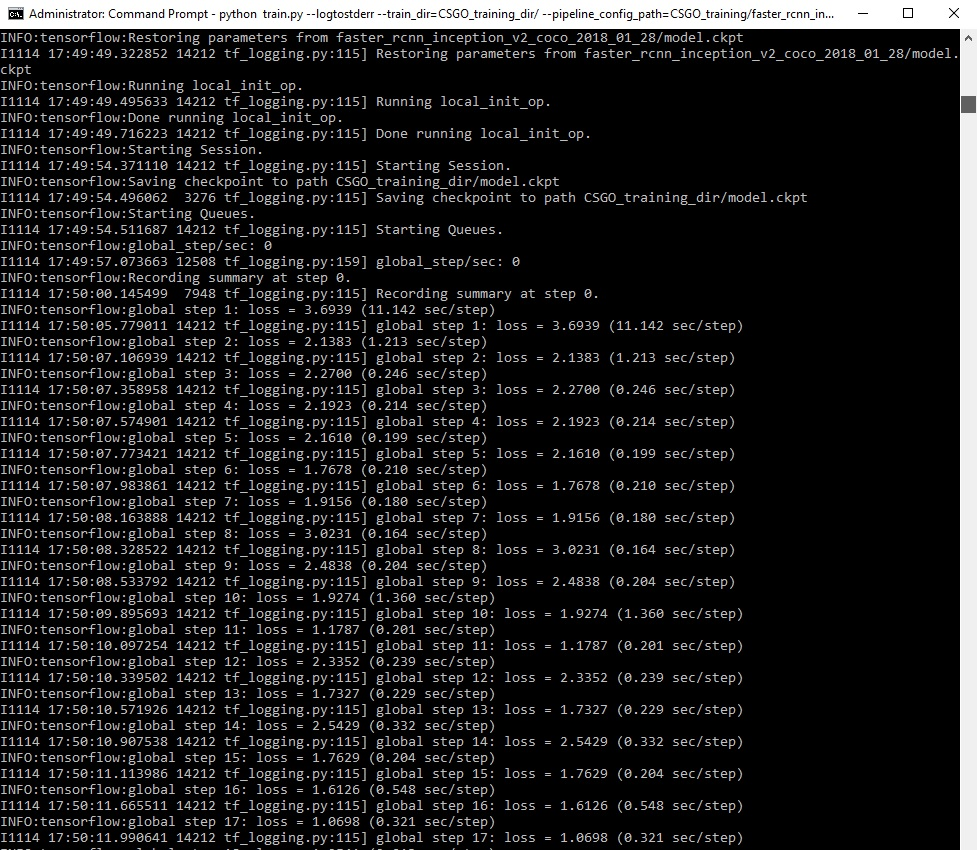In picture above, each step of training reports the loss. It will start high and get lower and lower as training progresses. For my training it started at about 2-3 and quickly dropped below 0.5. I recommend allowing your model to train until the loss consistently drops below 0.05, which may take about 30,000 steps, or about few hours (depends on how powerful your CPU or GPU is). The loss numbers may be different while different model is used. Also it depends from the objects you want to detect.

You can and you should view the progress of the training by using TensorBoard. To do this, open a new window of CMD and change to the C:\TensorFlow\research\object_detection directory (or directory you have) and issue the following command:
`C:\TensorFlow\research\object_detection>tensorboard --logdir=CSGO_training_dir`

This will create a local webpage on your local machine at YourPCName:6006, which can be viewed through a web browser. The TensorBoard page provides information and graphs that show how the training is progressing. One of the most important graph is the Loss graph, which shows the overall loss of the classifier over time: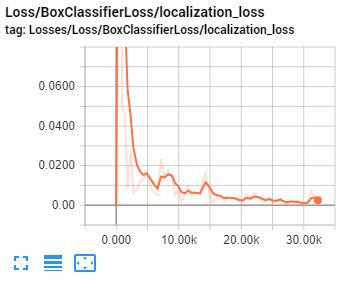The training routine periodically saves checkpoints about every ten minutes. You can terminate the training by pressing Ctrl+C while in the command prompt window. I usually wait until checkpoint has been saved to terminate the training. Then you can terminate training and start it later, and it will restart from the last saved checkpoint.

That’s all for this tutorial, in next tutorial checkpoint at the highest number of steps will be used to generate the frozen inference graph and test it out

# Part 4. TensorFlow Object Detection CSGO actual object detection

Welcome to part 6 of our TensorFlow Object Detection API tutorial series. In this part, we're going to export inference graph and detect our own custom objects.

#### Export Inference Graph:

Now when training is complete, the last step is to generate the frozen inference graph (our detection model). Copy export_inference_graph.py file and paste it to `/object_detection` folder, then from command promt issue the following command, where “XXXX” in “model.ckpt-XXXX” should be replaced with the highest-numbered .ckpt file in the training folder:

``````python export_inference_graph.py --input_type image_tensor --pipeline_config_path CSGO_training/faster_rcnn_inception_v2_coco.config --trained_checkpoint_prefix CSGO_training_dir/model.ckpt-XXXX --output_directory CSGO_inference_graph
``````

### Use our trained custom object detection classifier:

Above line creates a frozen_inference_graph.pb file in the /object_detection/CSGO_inference_graph folder. The .pb file contains the object detection classifier. Rename it to CSGO_frozen_inference_graph.pb and move it to your main working folder. Also take same labelmap file as you used for training, in my case I renamed it to CSGO_labelmap.pbtxt. Then I took `object_detection_tutorial_grabscreen_faster.py` code from my own 4th tutorial and renamed it to CSGO_object_detection.py and changed few lines, that it could work for us:

Changed line 39 to my frozen inference graph file.
`PATH_TO_FROZEN_GRAPH = 'CSGO_frozen_inference_graph.pb'`

Changed line 41 to my labelmap file.
`PATH_TO_LABELS = 'CSGO_labelmap.pbtxt'`

And lastly before running the Python scripts, you need to modify the line 42 NUM_CLASSES variable in the script to equal the number of classes we want to detect. I am using only 4 classes, so I changed it to 4:
`NUM_CLASSES = 4`

If everything is working properly, the object detector will initialize for about 10 (for GPU may take a little longer) seconds and then display a custom window size showing objects it’s detected in the image, in our case it's detecting players in CSGO game.

# Part 5. TensorFlow CS:GO custom object detection aim bot

Welcome to part 7 of our TensorFlow Object Detection API tutorial series. In this part, we're going to change our code, that we could find center of rectangles on our enemies, move our mouse to the center and shoot them.

In this tutorial we are working with same files as we used in 6th tutorial. To achieve desired goals for this tutorial we’ll need to add several lines to the code. At first we start with importing pyautogui library:

``````import pyautogui
``````

This library will be used to move our mouse in game. But some games may not allow you to move mouse, then you will need to start python script with administrator rights, same as I am doing for CSGO in my YouTube video tutorial.

Next we are changing defined monitor size line to following. We are doing this because we will use our window width and height in other places to calculate right coordinates for our game. So to avoid mistakes and not to write same values in many places, we are defining our window size accordingly:

``````width = 800
height = 640
monitor = {'top': 80, 'left': 0, 'width': width, 'height': height}
``````

Before moving to our main while loop we are defining new function, which we'll use to aim and shoot enemies. As you can see in following function, we are calculating y differently from x. In my YouTube tutorial we’ll see that when we are calculating y in same way as x, we are shooting above the head. So we are removing that difference dividing our desired screen height by 9 and adding it to standard y height.

``````def Shoot(mid_x, mid_y):
x = int(mid_x*width)
y = int(mid_y*height+height/9)
pyautogui.moveTo(x,y)
pyautogui.click()
``````

Next, we are improving our code, while working in our main while loop. So we create following for loop. At first we initialize array_ch array, where we will place all our ch objects. Then we are going through boxes array, and if we find our needed classes we check it for detection percentage. For example in our case classes[i] == 2 is equal to ch and if scores[i] >= 0.5 of this class is equal or more that 50 percent we assume that we detected our object. In this case we are taking boxes array numbers, where:
`boxes[i] – y axis upper start coordinates`
`boxes[i] – x axis left start coordinates`
`boxes[i] – y axis down start coordinates`
`boxes[i] – x axis right start coordinates`

While subtracting same axis start coordinates and dividing them by two we receive center of two axis. This way we can calculate center of our detected rectangle. And at the last line we are drawing a dot in a center:

``````array_ch = []
for i,b in enumerate(boxes):
if classes[i] == 2: # ch
if scores[i] >= 0.5:
mid_x = (boxes[i]+boxes[i])/2
mid_y = (boxes[i]+boxes[i])/2
array_ch.append([mid_x, mid_y])
cv2.circle(image_np,(int(mid_x*width),int(mid_y*height)), 3, (0,0,255), -1)
``````

These few line of code were only for one object, we do this for all four objects:

``````for i,b in enumerate(boxes):
if classes[i] == 2: # ch
if scores[i] >= 0.5:
mid_x = (boxes[i]+boxes[i])/2
mid_y = (boxes[i]+boxes[i])/2
array_ch.append([mid_x, mid_y])
cv2.circle(image_np,(int(mid_x*width),int(mid_y*height)), 3, (0,0,255), -1)
if classes[i] == 1: # c
if scores[i] >= 0.5:
mid_x = (boxes[i]+boxes[i])/2
mid_y = boxes[i] + (boxes[i]-boxes[i])/6
array_c.append([mid_x, mid_y])
cv2.circle(image_np,(int(mid_x*width),int(mid_y*height)), 3, (50,150,255), -1)
if classes[i] == 4: # th
if scores[i] >= 0.5:
mid_x = (boxes[i]+boxes[i])/2
mid_y = (boxes[i]+boxes[i])/2
array_th.append([mid_x, mid_y])
cv2.circle(image_np,(int(mid_x*width),int(mid_y*height)), 3, (0,0,255), -1)
if classes[i] == 3: # t
if scores[i] >= 0.5:
mid_x = (boxes[i]+boxes[i])/2
mid_y = boxes[i] + (boxes[i]-boxes[i])/6
array_t.append([mid_x, mid_y])
cv2.circle(image_np,(int(mid_x*width),int(mid_y*height)), 3, (50,150,255), -1)
``````

After this we are making shooting function. So as a team = "t" we choose who we will be shooting at, at this case we are trying to shoot terrorists. So at first we check if we have detected terrorists heads, if we have detected at least one head, we call `Shoot(mid_x, mid_y)` function with needed coordinates. If we don't have heads detected we check maybe we have detected terrorists bodies, if we did, we call the same shooting function. Otherwise we don't call Shooting function.

``````team = "t"
if team == "c":
if len(array_ch) > 0:
Shoot(array_ch, array_ch)
if len(array_ch) == 0 and len(array_c) > 0:
Shoot(array_c, array_c)
if team == "t":
if len(array_th) > 0:
Shoot(array_th, array_th)
if len(array_th) == 0 and len(array_t) > 0:
Shoot(array_t, array_t)
``````

If we would like to shoot to counter-terrorists we change "t" to "c" at first line.

This was only a short explanation of code, full code you can download from above files. In my YouTube video you can see how my CSGO aim bot model is working. For now, I am really disappointed about our FPS, because no one can play at these numbers... But I am glad that our bot can target to enemies quite accurate and shoot them. So maybe for next tutorial I will think what we could do to make it work faster for us.

# Part 6. TensorFlow CSGO custom object detection faster aim bot

Welcome to part 8 of the TensorFlow Object Detection API tutorial series. In this tutorial I will show you how to export newly trained model and we'll test it out.

So in previous tutorial we made a final working model, which shoots enemies, but our FPS were really slow, so I decided to try training another model, so that's what we will talk about in this tutorial.

I used almost all the same files from 5th tutorial part, so if you don't have them yet you can clone my GitHub repository. In this part I am not covering how to label pictures, generate tfrecord or configure your training files. I already did this on my 5th tutorial. In this tutorial I will cover only this, which were not covered before.

At first trained model in 5th tutorial I used faster_rcnn_inception_v2_coco model, now I decided to train ssdlite_mobilenet_v2_coco, this model detects objects 21% worse but it is 53% faster, so I decided give it a try. Here is the link of all models, so download one if you decided to train model by yourself.

This time when I tries to use train.py file it said that I am using wrong training method, and offered to use model_main.py file. So I uploaded it if someone has problems finding it. I faced some problems when I tried to start it training the model, there were some error but I didn't made a note about them so I can't tell it exactly, so if you face errors, write it on YouTube comments, we'll try to solve it.

When training new model I was using same file structure, so you will need only to update ssdlite_mobilenet_v2_coco.config file and download your pretrained model. From TensorFlow/research/object_detection folder continue with following line in cmd:

``````python model_main.py --alsologtostderr --model_dir=CSGO_training_dir/ --pipeline_config_path=CSGO_training/ssdlite_mobilenet_v2_coco.config
``````

When training model, it will not show steps as it was doing in 5th tutorial, but training routine periodically saves model checkpoints about every ten minutes to CSGO_training_dir directory. So you should check how your training is going in TensorFlow tensorboard, you can do so with following command:

``````C:\TensorFlow\research\object_detection>tensorboard --logdir=CSGO_training_dir
``````

I was training my model since I saw that my loss curve stopped dropping. It took for me close to 24 hours and did around 21k training steps: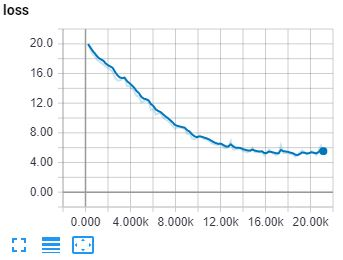Then I used same export_inference_graph.py as we used in 6th tutorial. From command promt issued the following command, where “XXXX” in “model.ckpt-XXXX” should be replaced with the highest-numbered .ckpt file in the training folder:

``````python export_inference_graph.py --input_type image_tensor --pipeline_config_path CSGO_training/ssdlite_mobilenet_v2_coco.config --trained_checkpoint_prefix CSGO_training_dir/model.ckpt-XXXX --output_directory CSGO_inference_graph
``````

In final step, we tooks all files from my 7th tutorial and replaced CSGO_frozen_inference_graph.pb file with newly trained inference_graph.

Next we tried to play CS:GO and I let my bot to shoot enemies, you can check this out on my YouTube video.

That’s all for this tutorial. With new model I didn't solved FPS problem, it improved performance slightly but not that we could play our game. So for future work I decided to learn doing stuff on multiprocessing and run our code processes in parallel. So in next tutorial I will be doing stuff with multiprocessing.

# Part 7. Grab screen with multiprocessings

Welcome everyone to part 9 of our TensorFlow object detection API series. This tutorial will be a little different from previous tutorials.

In 8 part I told that I will be working with python multiprocessing to make code work in parallel with other processes. So I spent hours of learning how to use multiprocessing (was not using it before).

So I copied whole code from my second tutorial and removed `screen_recordPIL` and `screen_grab` functions. Left only to work with `screen_recordMSS` function. This function we can divide into two parts where we grab screen and where we show our grabbed screen. So this mean we will need to create two processes.

At first I divide whole code into two parts, first part we will call GRABMSS_screen. Next we need to put whole code into while loop, that it would run over and over. When we have our screen, we call `q.put_nowait(img)` command where we put our image into shared queue, and with following line `q.join()` we are saying wait since img will be copied to queue.

``````def GRABMSS_screen(q):
while True:
# Get raw pixels from the screen, save it to a Numpy array
img = numpy.array(sct.grab(monitor))
# To get real color we do this:
#img = cv2.cvtColor(img, cv2.COLOR_BGR2RGB)
q.put_nowait(img)
q.join()
``````

Second function we will call SHOWMSS_screen. This function also will run in a while loop, and we always check if our queue is not empty. When we have something in queue we call `q.get_nowait()` command which takes everything from queue, and with `q.task_done()` we are locking the process, not to interrupt queue if we didn't finished picking up all data. After that we do same things as before, showing grabbed image and measuring FPS.

``````def SHOWMSS_screen(q):
global fps, start_time
while True:
if not q.empty():
img = q.get_nowait()
# To get real color we do this:
img = cv2.cvtColor(img, cv2.COLOR_BGR2RGB)
# Display the picture
cv2.imshow(title, cv2.cvtColor(img, cv2.COLOR_BGR2RGB))
# Display the picture in grayscale
fps+=1
TIME = time.time() - start_time
if (TIME) >= display_time :
print("FPS: ", fps / (TIME))
fps = 0
start_time = time.time()
# Press "q" to quit
if cv2.waitKey(25) & 0xFF == ord("q"):
cv2.destroyAllWindows()
break
``````

Right now, we have two different functions, we will use them in parallel processes.

If we want to run our code in multiprocessing we must begin our code with `if __name__=="__main__":` and we must run python script from command prompt elsewise if we'll run it from python shell, we won't get any prints, which we need here to measure FPS. So our full 3rd code part looks like this:

``````if __name__=="__main__":
# Queue
q = multiprocessing.JoinableQueue()

# creating new processes
p1 = multiprocessing.Process(target=GRABMSS_screen, args=(q, ))
p2 = multiprocessing.Process(target=SHOWMSS_screen, args=(q, ))

# starting our processes
p1.start()
p2.start()
``````

More about python multiprocessing and queues you can learn on this link. Short code explanation: We begin with creating a chared queue:

``````# Queue
q = multiprocessing.JoinableQueue()
``````

With following lines we are creating p1 and p2 processes which will run in background. p1 function will call GRABMSS_screen() function and p2 will call SHOWMSS_screen() function. As an argument for these functions we must give arguments, we give q there.

``````# creating new processes
p1 = multiprocessing.Process(target=GRABMSS_screen, args=(q, ))
p2 = multiprocessing.Process(target=SHOWMSS_screen, args=(q, ))
``````

Final step is to start our processes, after these commands our grab screen function will run in background.

``````# starting our processes
p1.start()
p2.start()
``````

For comparison I ran old code without multiprocessing and with multiprocessing. Here is results without multiprocessing: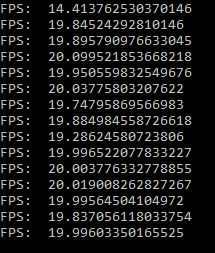We can see that average is about 19-20 FPS. Here is results with multiprocessing: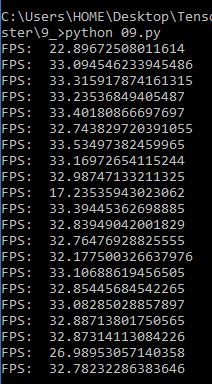We can see that average is about 32 FPS. So our final result is that our grab screen improved in around 50%. I would like like to impove it more, but for now I don't have ideas how to do that. Anyway results are much better than before !

# Part 8. Final TensorFlow aim bot with multiprocessing

Welcome everyone to part 10 of our TensorFlow object detection API tutorial series. For now this will be the final tutorial of this CSGO aim bot video series, because right now I spent too much time on this tutorial. I managed to get best performance as I can for now, for further improvements I would need to investigate time to another detection methods what I will do in future.

Before continuing on this tutorial I should mention, that I updated 9th tutorial code before merging it with CSGO TensorFlow detection code. So I updated 9th tutorial, added one more file in which we are grabbing screen using multiprocessing pipes. Comparing with multiprocessing queues performance is the same (33FPS), but I wanted to test different methods to share data between processes. Adding to multiprocessing pipes, they use communication one to one, and queues may be used as many to many.

Continuing on this tutorial, I am not going to code explanation in this text tutorial part. I simply divided whole code into 3 parts: grabbing screen, making tensorflow detection and showing the screen. All these 3 parts were moved to multiprocessing processes.

Here is the final code:

``````# # Imports
import multiprocessing
from multiprocessing import Pipe
import time
import cv2
import mss
import numpy as np
import os
import sys
os.environ['CUDA_VISIBLE_DEVICES'] = '0'
import tensorflow as tf
from distutils.version import StrictVersion
from collections import defaultdict
from io import StringIO
import pyautogui

# title of our window
title = "FPS benchmark"
# set start time to current time
start_time = time.time()
# displays the frame rate every 2 second
display_time = 2
# Set primarry FPS to 0
fps = 0
# Load mss library as sct
sct = mss.mss()
# Set monitor size to capture to MSS
width = 800
height = 640

monitor = {"top": 80, "left": 0, "width": width, "height": height}

# ## Env setup
from object_detection.utils import ops as utils_ops
from object_detection.utils import label_map_util
from object_detection.utils import visualization_utils as vis_util

# # Model preparation
PATH_TO_FROZEN_GRAPH = 'CSGO_frozen_inference_graph.pb'
# List of the strings that is used to add correct label for each box.
PATH_TO_LABELS = 'CSGO_labelmap.pbtxt'
NUM_CLASSES = 4

# ## Load a (frozen) Tensorflow model into memory.
categories = label_map_util.convert_label_map_to_categories(label_map, max_num_classes=NUM_CLASSES, use_display_name=True)
category_index = label_map_util.create_category_index(categories)

detection_graph = tf.Graph()
with detection_graph.as_default():
od_graph_def = tf.GraphDef()
with tf.gfile.GFile(PATH_TO_FROZEN_GRAPH, 'rb') as fid:
od_graph_def.ParseFromString(serialized_graph)
tf.import_graph_def(od_graph_def, name='')

def Shoot(mid_x, mid_y):
x = int(mid_x*width)
#y = int(mid_y*height)
y = int(mid_y*height+height/9)
pyautogui.moveTo(x,y)
pyautogui.click()

def grab_screen(p_input):
while True:
#Grab screen image
img = np.array(sct.grab(monitor))
img = cv2.cvtColor(img, cv2.COLOR_BGR2RGB)
img = cv2.cvtColor(img, cv2.COLOR_BGR2RGB)

# Put image from pipe
p_input.send(img)

def TensorflowDetection(p_output, p_input2):
# Detection
with detection_graph.as_default():
with tf.Session(graph=detection_graph) as sess:
while True:
# Get image from pipe
image_np = p_output.recv()
# Expand dimensions since the model expects images to have shape: [1, None, None, 3]
image_np_expanded = np.expand_dims(image_np, axis=0)
# Actual detection.
image_tensor = detection_graph.get_tensor_by_name('image_tensor:0')
boxes = detection_graph.get_tensor_by_name('detection_boxes:0')
scores = detection_graph.get_tensor_by_name('detection_scores:0')
classes = detection_graph.get_tensor_by_name('detection_classes:0')
num_detections = detection_graph.get_tensor_by_name('num_detections:0')
# Visualization of the results of a detection.
(boxes, scores, classes, num_detections) = sess.run(
[boxes, scores, classes, num_detections],
feed_dict={image_tensor: image_np_expanded})
vis_util.visualize_boxes_and_labels_on_image_array(
image_np,
np.squeeze(boxes),
np.squeeze(classes).astype(np.int32),
np.squeeze(scores),
category_index,
use_normalized_coordinates=True,
line_thickness=2)

# Send detection image to pipe2
p_input2.send(image_np)

array_ch = []
array_c = []
array_th = []
array_t = []
for i,b in enumerate(boxes):
if classes[i] == 2: # ch
if scores[i] >= 0.5:
mid_x = (boxes[i]+boxes[i])/2
mid_y = (boxes[i]+boxes[i])/2
array_ch.append([mid_x, mid_y])
cv2.circle(image_np,(int(mid_x*width),int(mid_y*height)), 3, (0,0,255), -1)
if classes[i] == 1: # c
if scores[i] >= 0.5:
mid_x = (boxes[i]+boxes[i])/2
mid_y = boxes[i] + (boxes[i]-boxes[i])/6
array_c.append([mid_x, mid_y])
cv2.circle(image_np,(int(mid_x*width),int(mid_y*height)), 3, (50,150,255), -1)
if classes[i] == 4: # th
if scores[i] >= 0.5:
mid_x = (boxes[i]+boxes[i])/2
mid_y = (boxes[i]+boxes[i])/2
array_th.append([mid_x, mid_y])
cv2.circle(image_np,(int(mid_x*width),int(mid_y*height)), 3, (0,0,255), -1)
if classes[i] == 3: # t
if scores[i] >= 0.5:
mid_x = (boxes[i]+boxes[i])/2
mid_y = boxes[i] + (boxes[i]-boxes[i])/6
array_t.append([mid_x, mid_y])
cv2.circle(image_np,(int(mid_x*width),int(mid_y*height)), 3, (50,150,255), -1)

team = "c" # shooting target
if team == "c":
if len(array_ch) > 0:
Shoot(array_ch, array_ch)
if len(array_ch) == 0 and len(array_c) > 0:
Shoot(array_c, array_c)
if team == "t":
if len(array_th) > 0:
Shoot(array_th, array_th)
if len(array_th) == 0 and len(array_t) > 0:
Shoot(array_t, array_t)

def Show_image(p_output2):
global start_time, fps
while True:
image_np = p_output2.recv()
# Show image with detection
cv2.imshow(title, image_np)
# Bellow we calculate our FPS
fps+=1
TIME = time.time() - start_time
if (TIME) >= display_time :
print("FPS: ", fps / (TIME))
fps = 0
start_time = time.time()
# Press "q" to quit
if cv2.waitKey(25) & 0xFF == ord("q"):
cv2.destroyAllWindows()
break

if __name__=="__main__":
# Pipes
p_output, p_input = Pipe()
p_output2, p_input2 = Pipe()

# creating new processes
p1 = multiprocessing.Process(target=grab_screen, args=(p_input,))
p2 = multiprocessing.Process(target=TensorflowDetection, args=(p_output,p_input2,))
p3 = multiprocessing.Process(target=Show_image, args=(p_output2,))

# starting our processes
p1.start()
p2.start()
p3.start()
``````

As a final result, I was quite happy that we can achieve more that 20 FPS. But when tensorflow receives images where we detect enemies here comes the bottleneck. FPS drops to 4-5 frames per seconds, and it becomes impossible to play this game for our bot. So in future I may come back to this project, when I find methods, how to detect our enemies faster. There is a way to use YOLO object detection model, it's quite fast and accurate but it's harder to implement (for now).

Anyway I think I spent a lot of time working on this project, right now I will move to other more benefit project. In nearest future I am planning to make tutorial how to crack CAPTCHA's, how to use SELENIUM to make web surfing bots or AI forex trading bot.

### Original text version of tutorial you can visit here.

Get A Weekly Email With Trending Projects For These Topics
No Spam. Unsubscribe easily at any time.
Python (807,238
Tutorials (23,565
Tensorflow (22,344
Label (11,601
Gpu (9,391
Object Detection (3,882
Cudnn (953
Tensorflow Models (408
Pil (115
Tensorflow Gpu (62
Detection Api (13